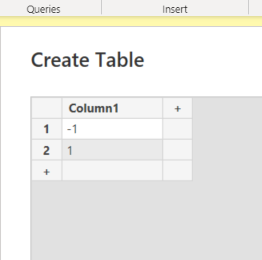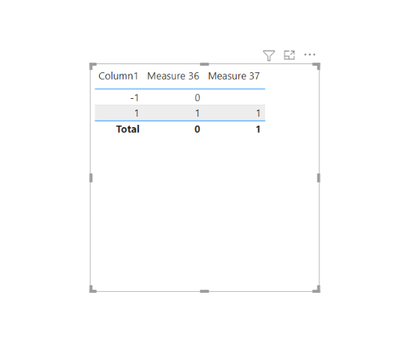cancel
Showing results for
Did you mean:Helper III

## Should be easy fix I am just baffled, DAX to change values from negative to zeros

Hi All,

I have this measure...

Free Stock Value =
Sum('ABC'[Quantity]) - Sum('DEF'[Outstanding Quantity])

Any way I can get this measure to change negative values returned into 0's?

I've used...

IF(Free Stock Value < 0, 0, Free Stock Value)

And it works fine but the total stays the exact same and doesn't add up properly it still assumes there is negative values present.

Any help would be greatly appricated!

Manuy Thanks,

Taylor
1 ACCEPTED SOLUTIONSuper User

Hi,

I recommend reading this article by sqlbi to understand what is going on here: Obtaining accurate totals in DAX - SQLBI

Basically the totals work in mysterious ways and even if your measure would work on a row level it will not work at total level. E.g.Measure 36 = IF(SELECTEDVALUE('Table (20)'[Column1])<0,0, SUM('Table (20)'[Column1]))
^^ won't work

Measure 37 = SUMX(FILTER('Table (20)', 'Table (20)'[Column1] >= 0), 'Table (20)'[Column1])
^^ will workI hope this post helps to solve your issue and if it does consider accepting it as a solution and giving the post a thumbs up!

Proud to be a Super User!

4 REPLIES 4Community Champion

when you say negative Free Stock Value, negative under what context?Helper III

Hi @FreemanZ,

Negative e.g. I subtract the 2 values away from eachother. Both numbers in the columns are always going to be positive so the new number in the measure column could possible be negative and I want those negatives to display zero instead of displaying as the negative number.

I am trying to work out free stock so....

Quantity = Stock currently sitting

Outstanding = Stock soldSuper User

Hi,

I recommend reading this article by sqlbi to understand what is going on here: Obtaining accurate totals in DAX - SQLBI

Basically the totals work in mysterious ways and even if your measure would work on a row level it will not work at total level. E.g.Measure 36 = IF(SELECTEDVALUE('Table (20)'[Column1])<0,0, SUM('Table (20)'[Column1]))
^^ won't work

Measure 37 = SUMX(FILTER('Table (20)', 'Table (20)'[Column1] >= 0), 'Table (20)'[Column1])
^^ will workI hope this post helps to solve your issue and if it does consider accepting it as a solution and giving the post a thumbs up!

Proud to be a Super User!Helper III

Nice @ValtteriN I will give it a read! Many Thanks!